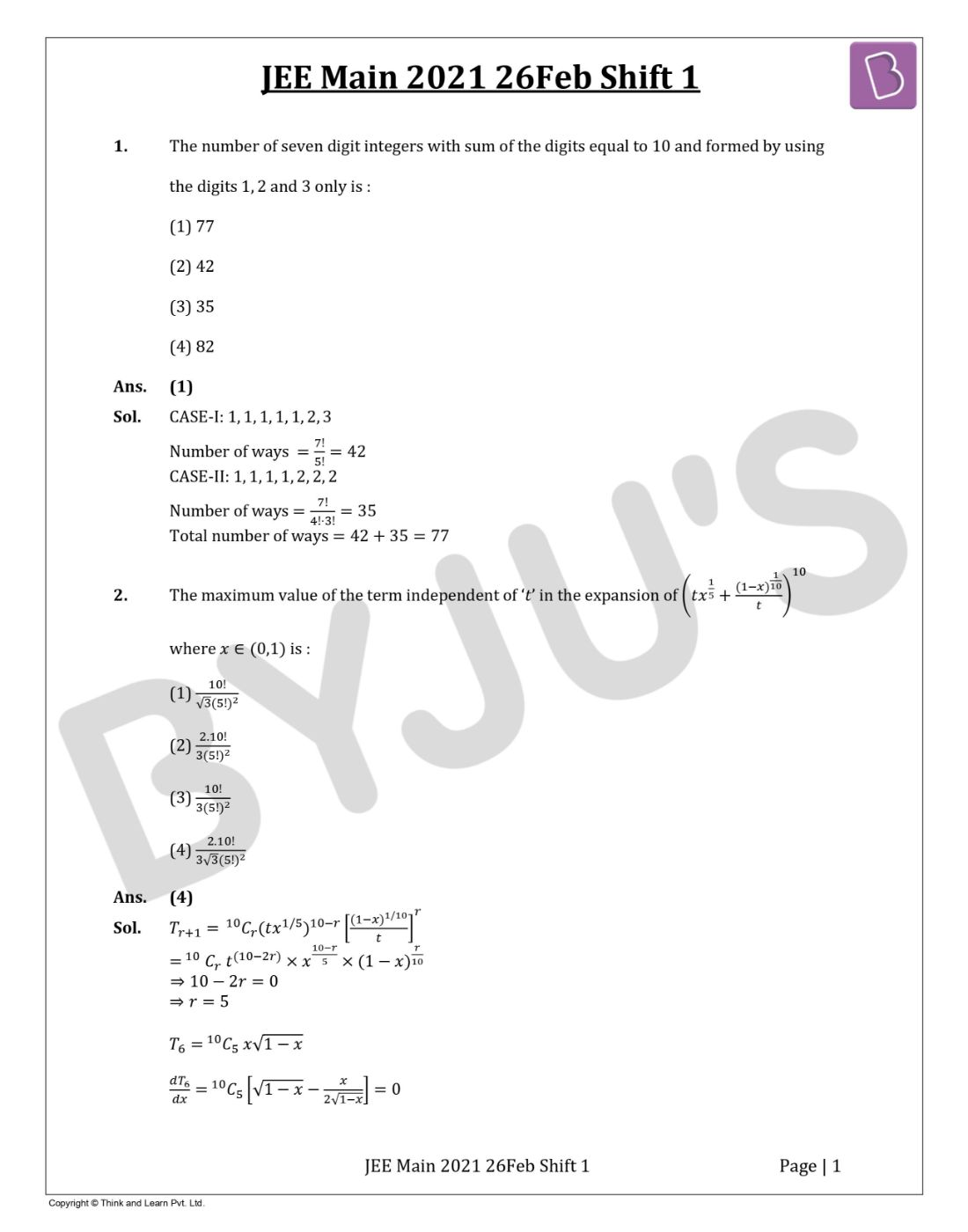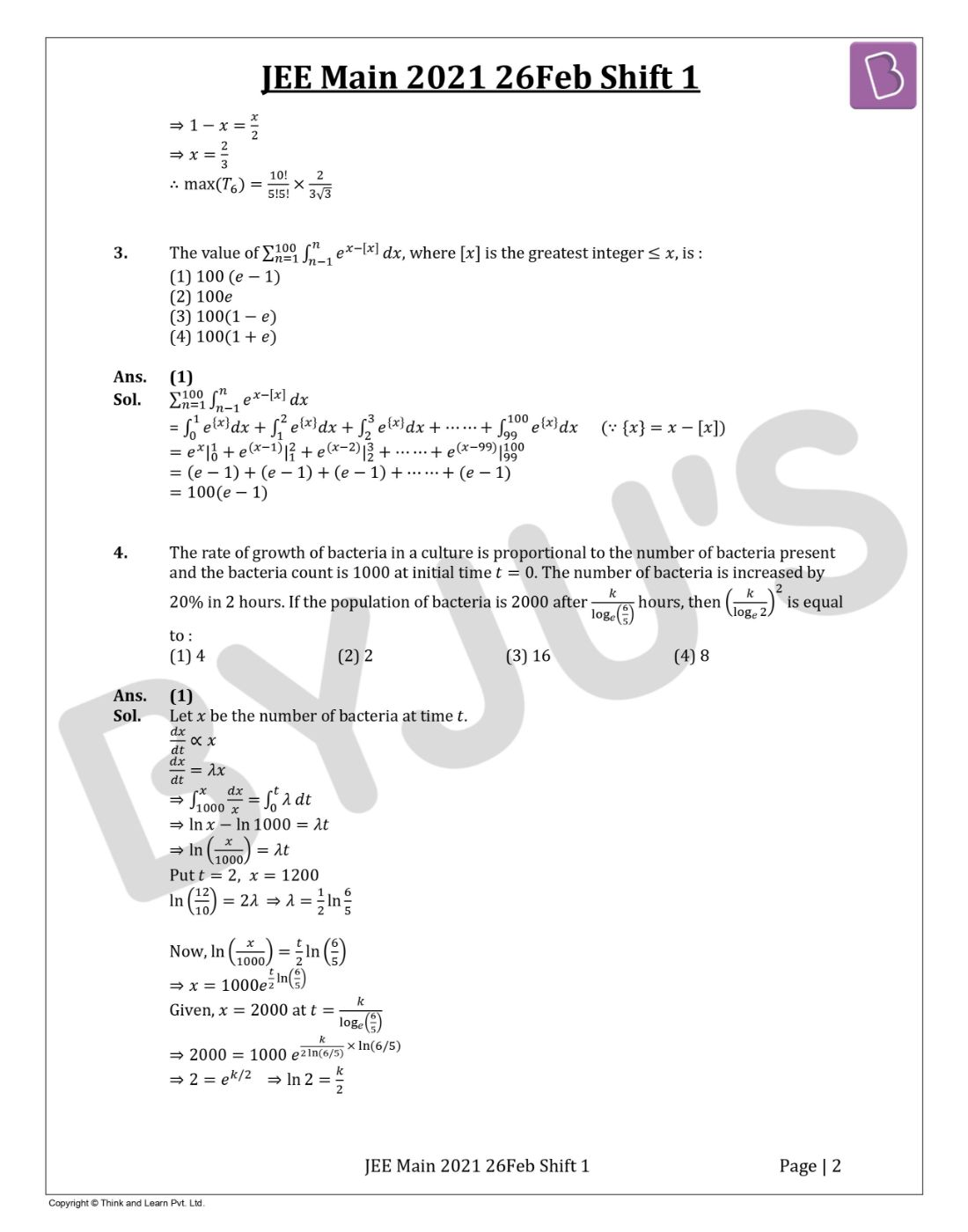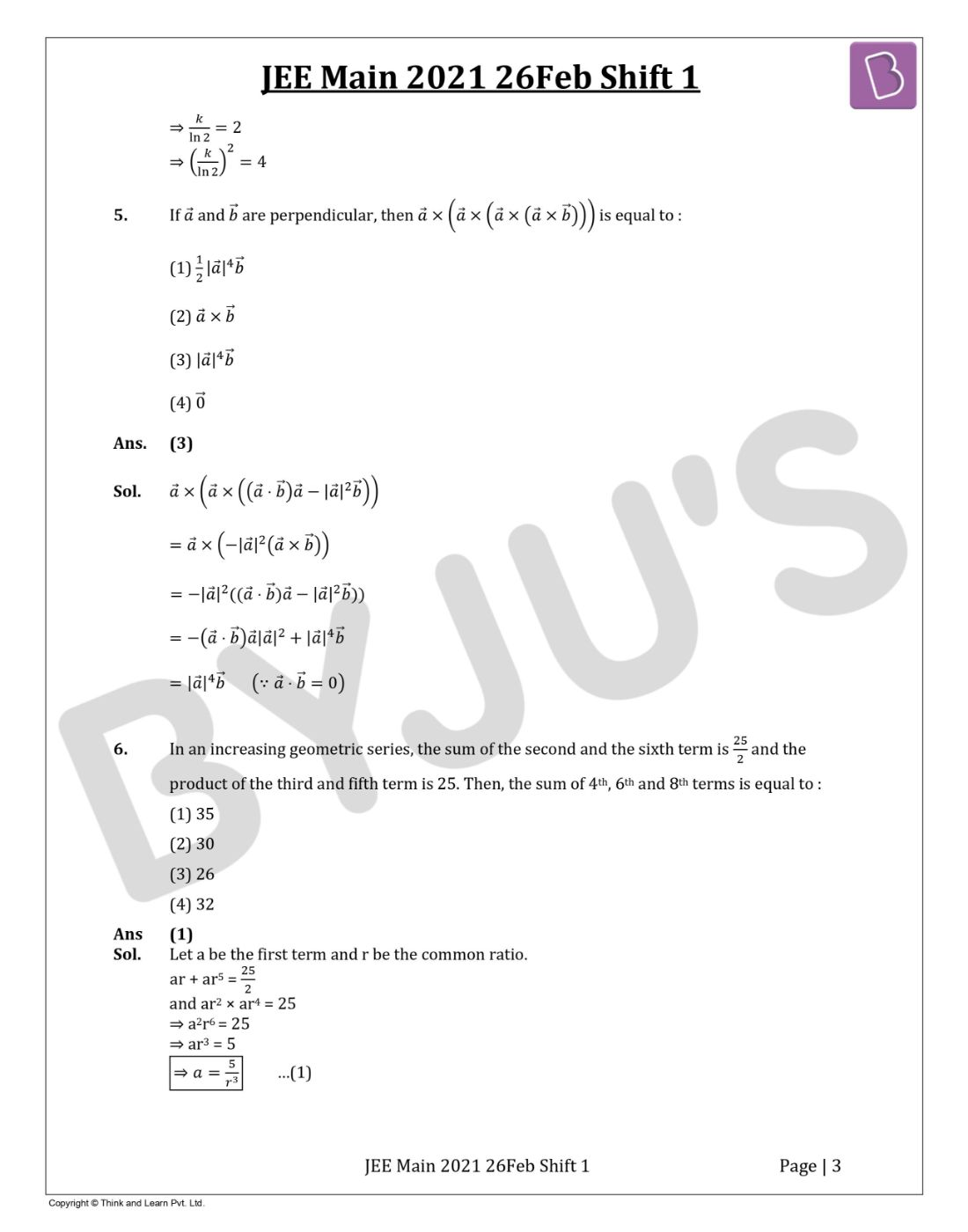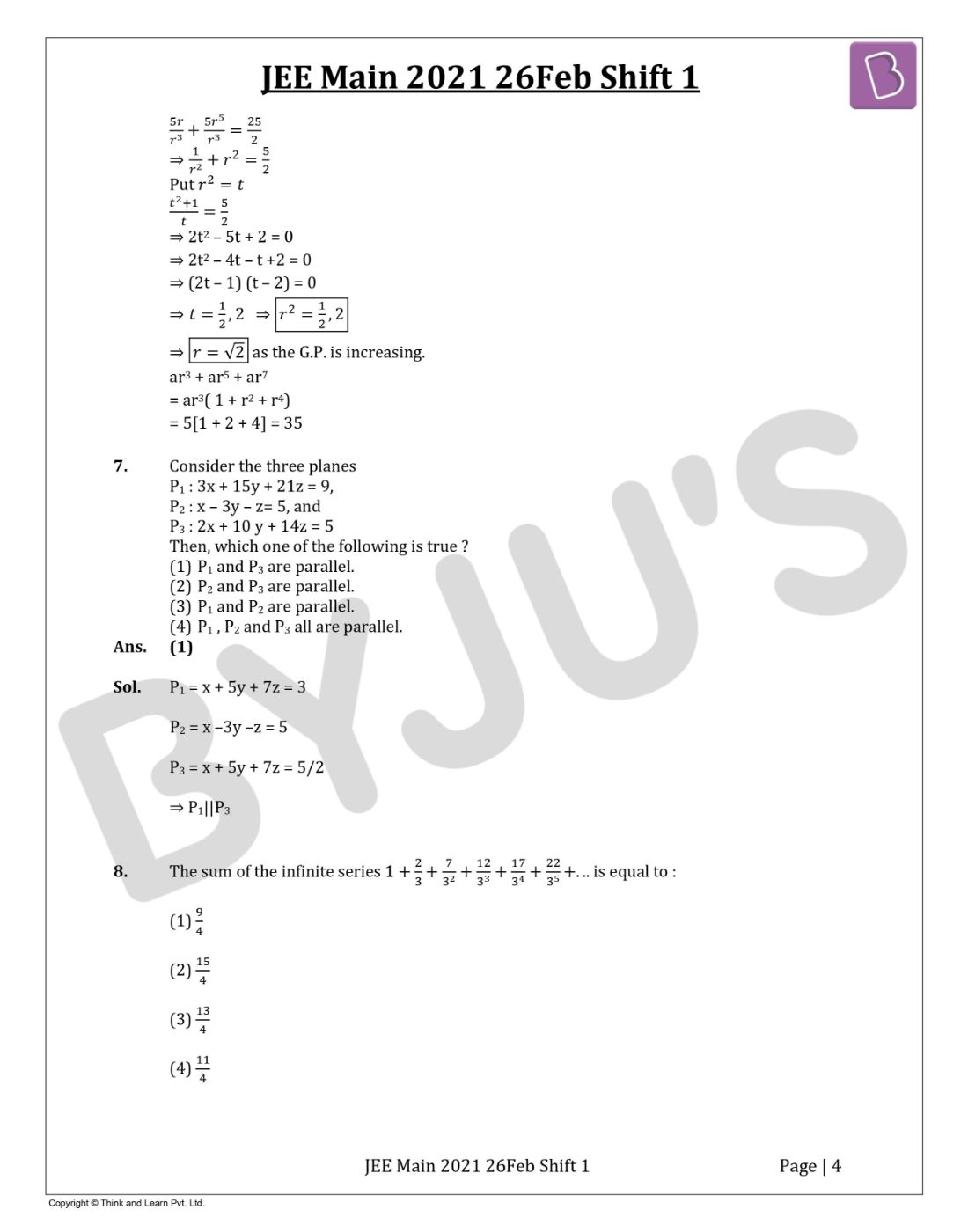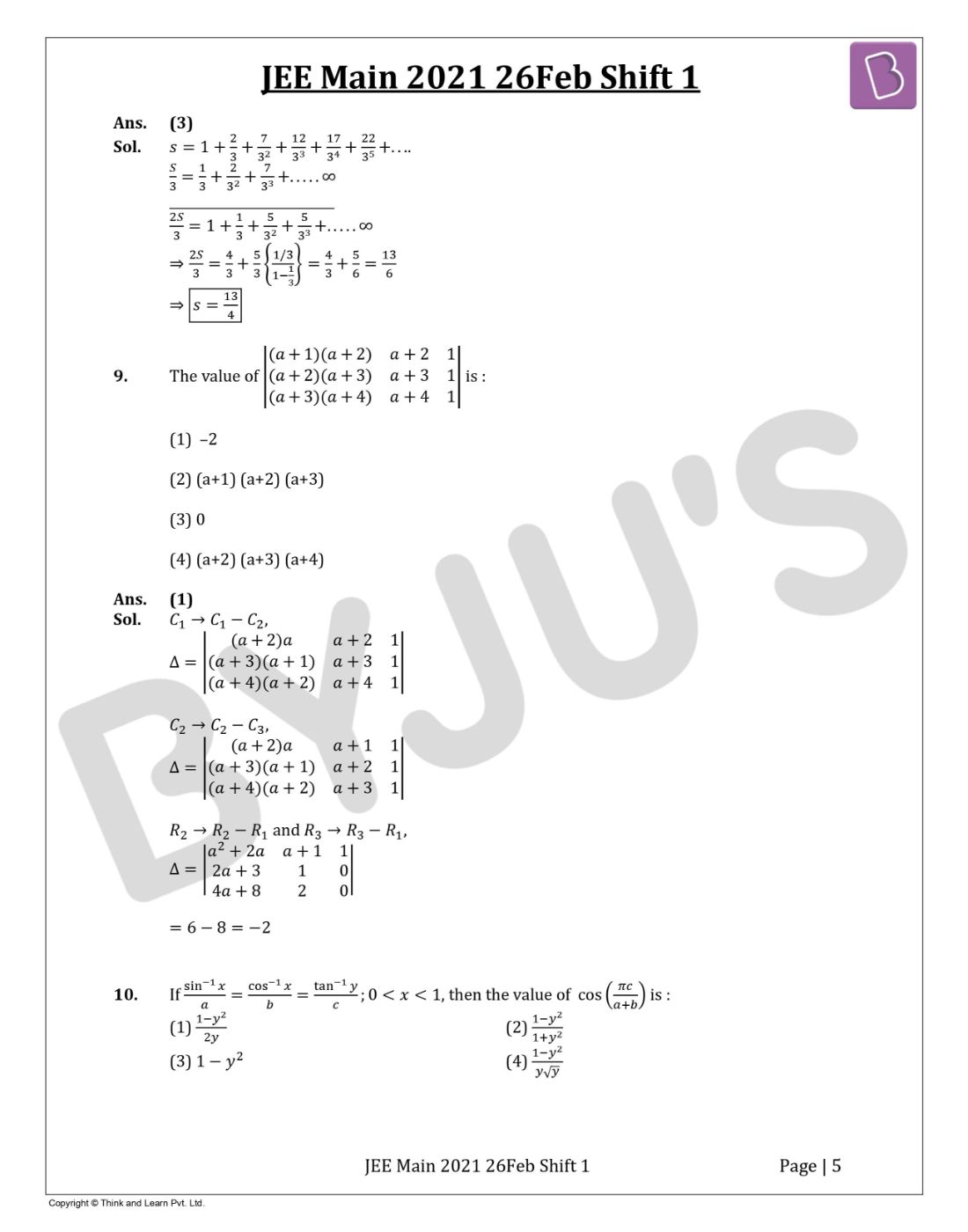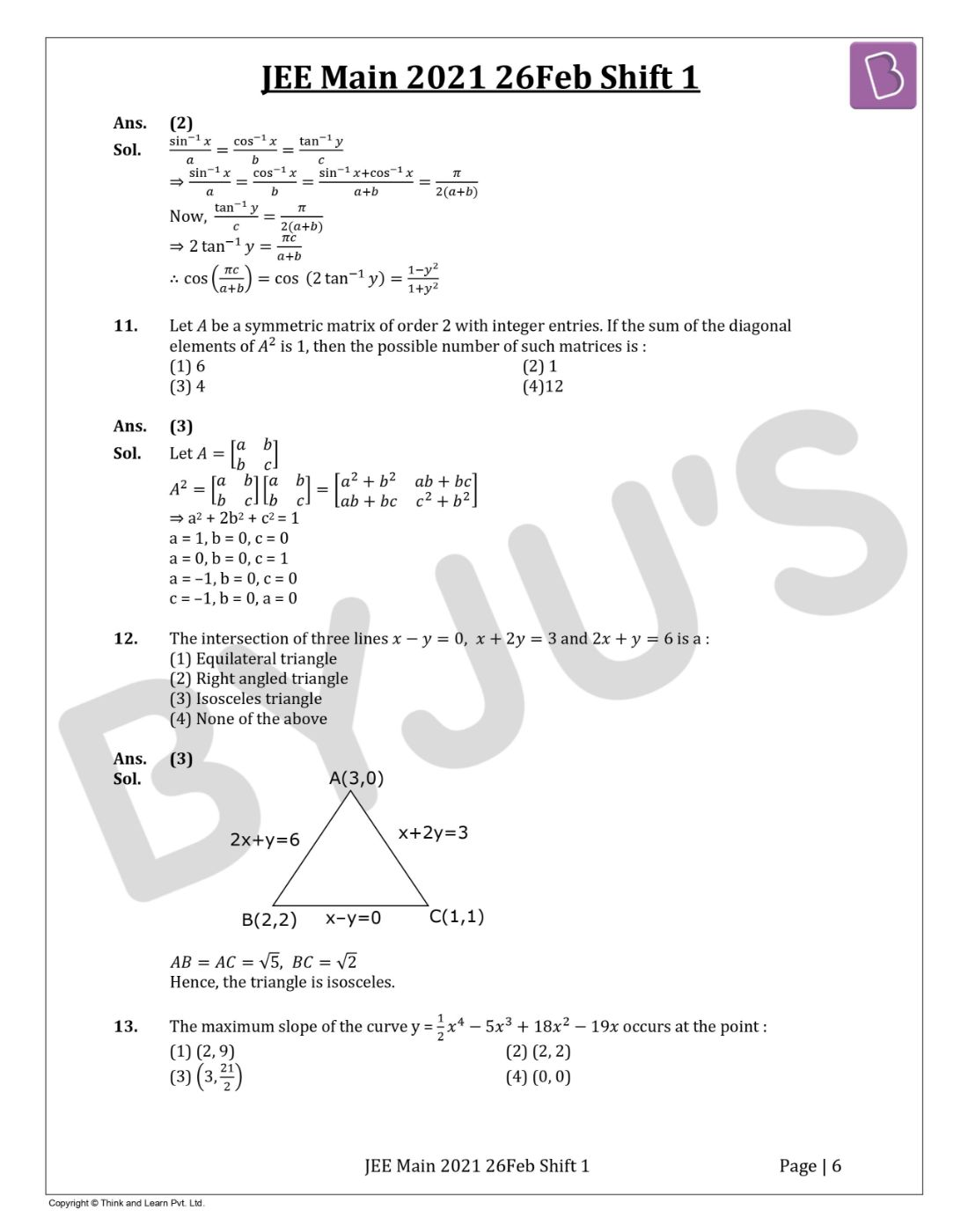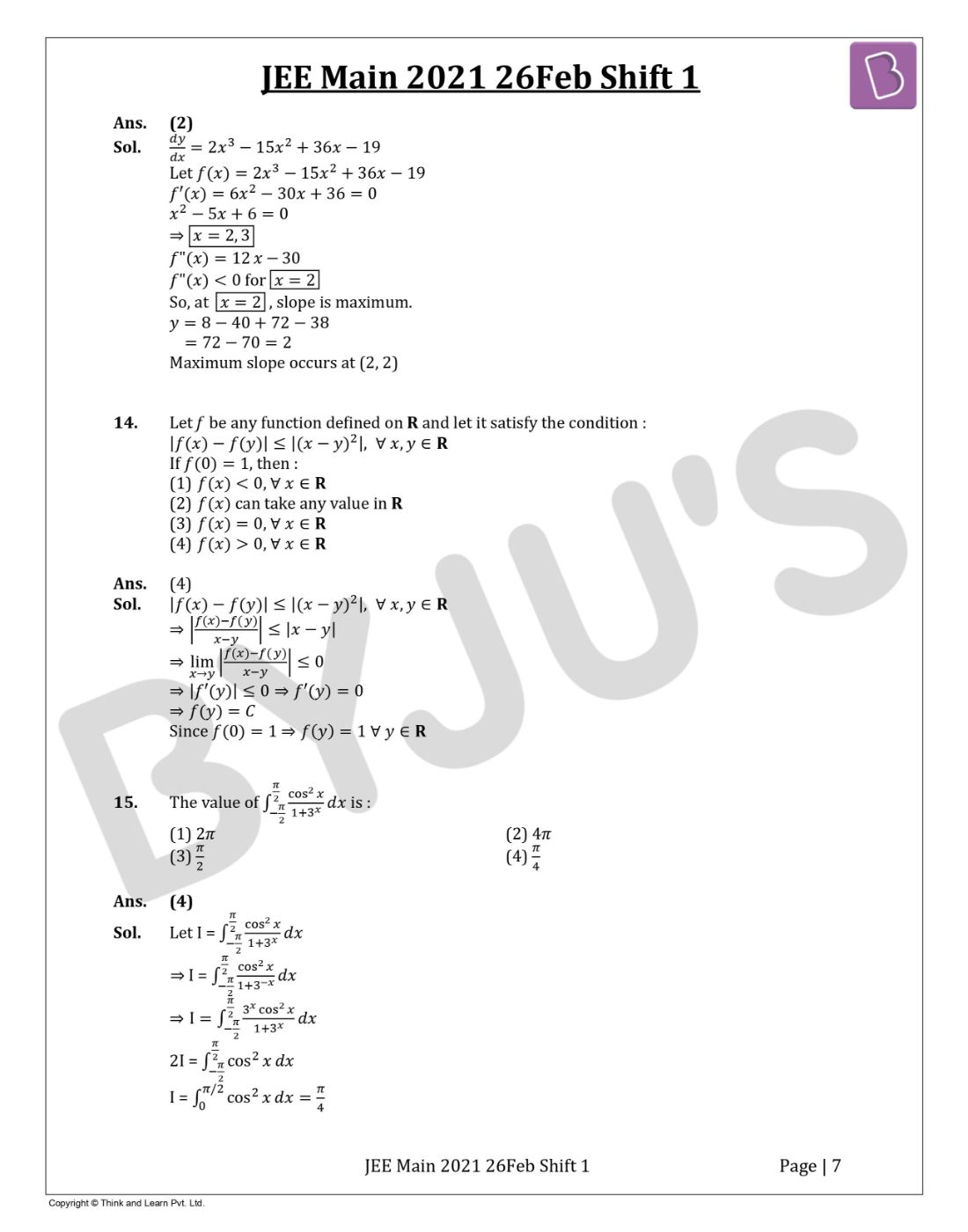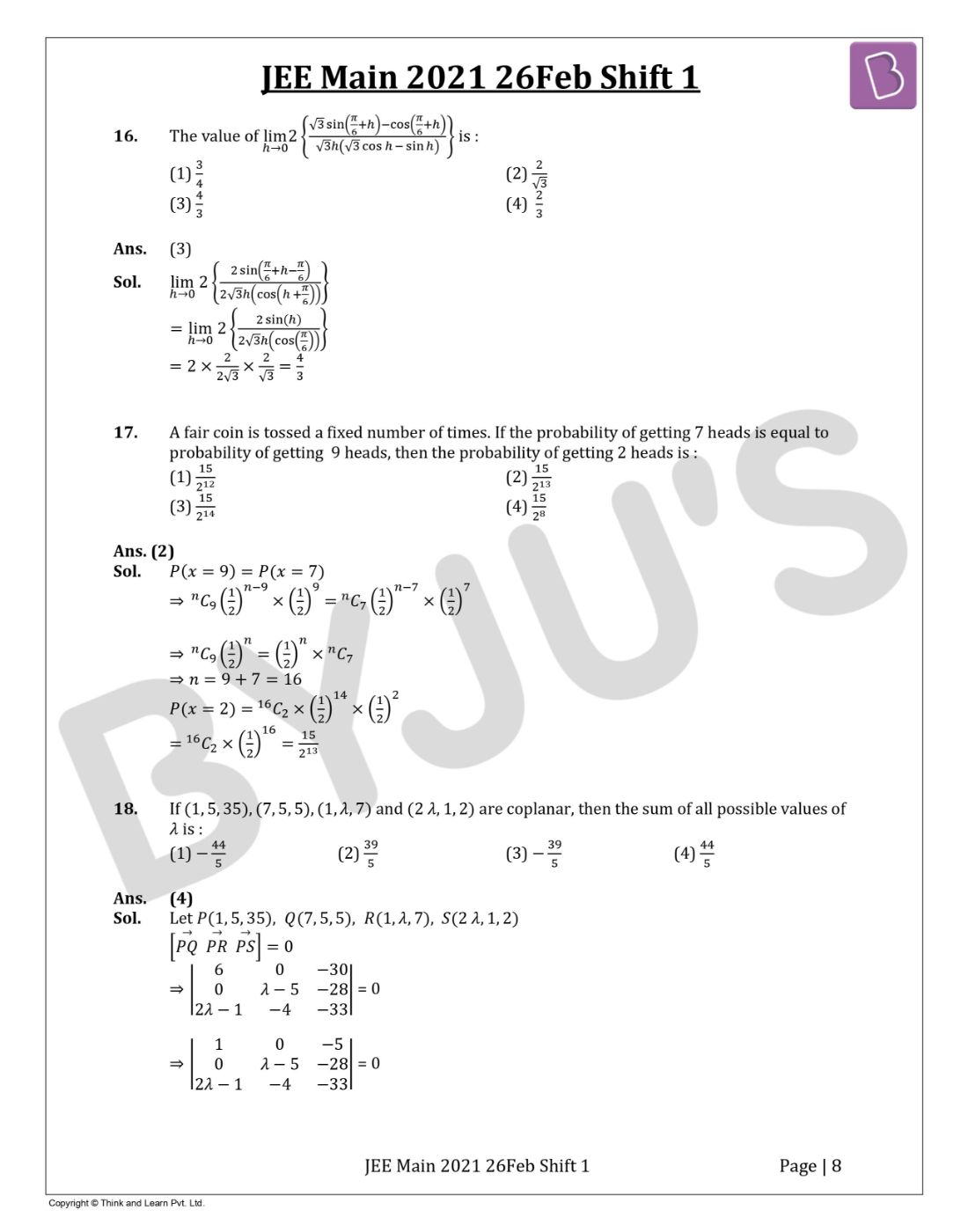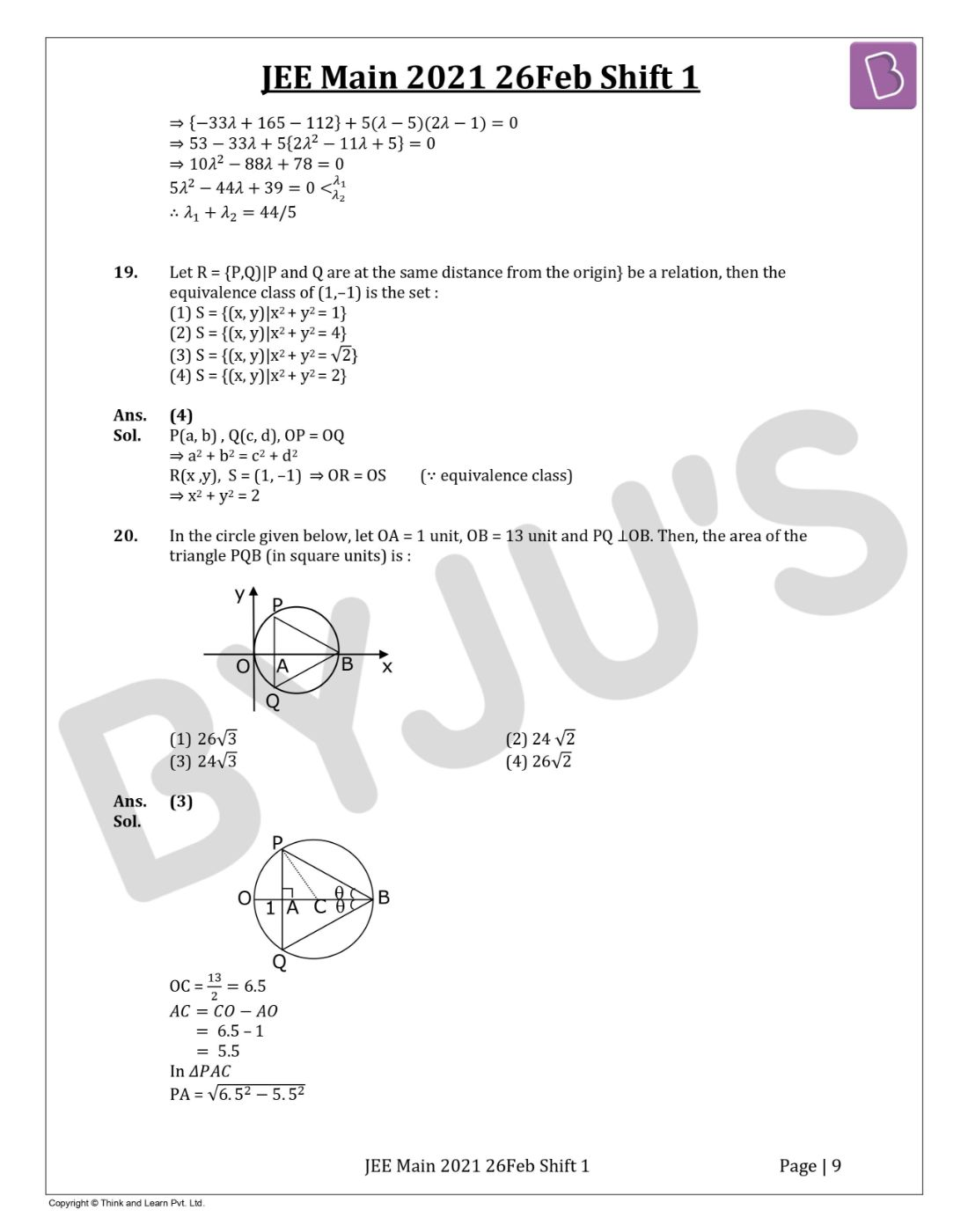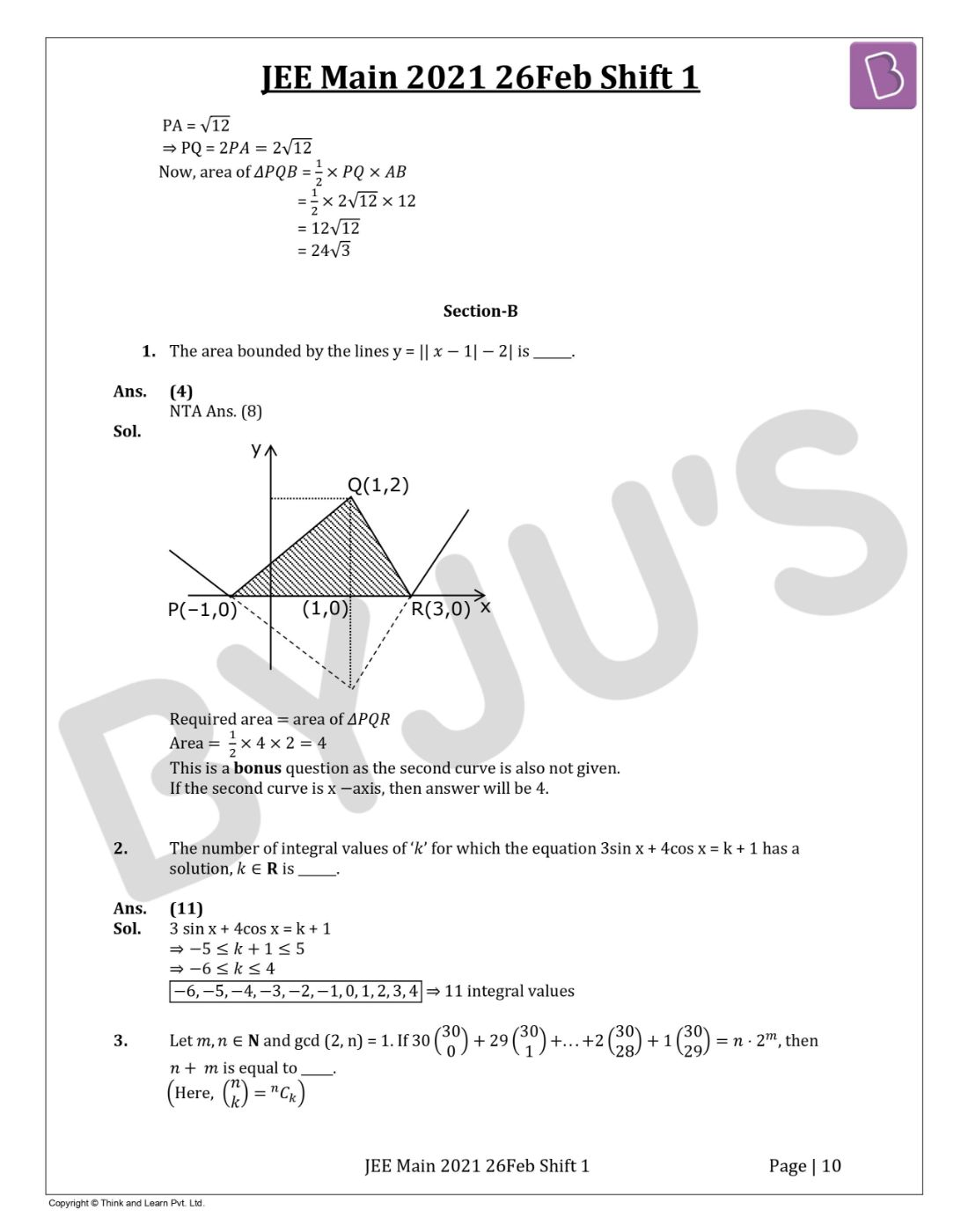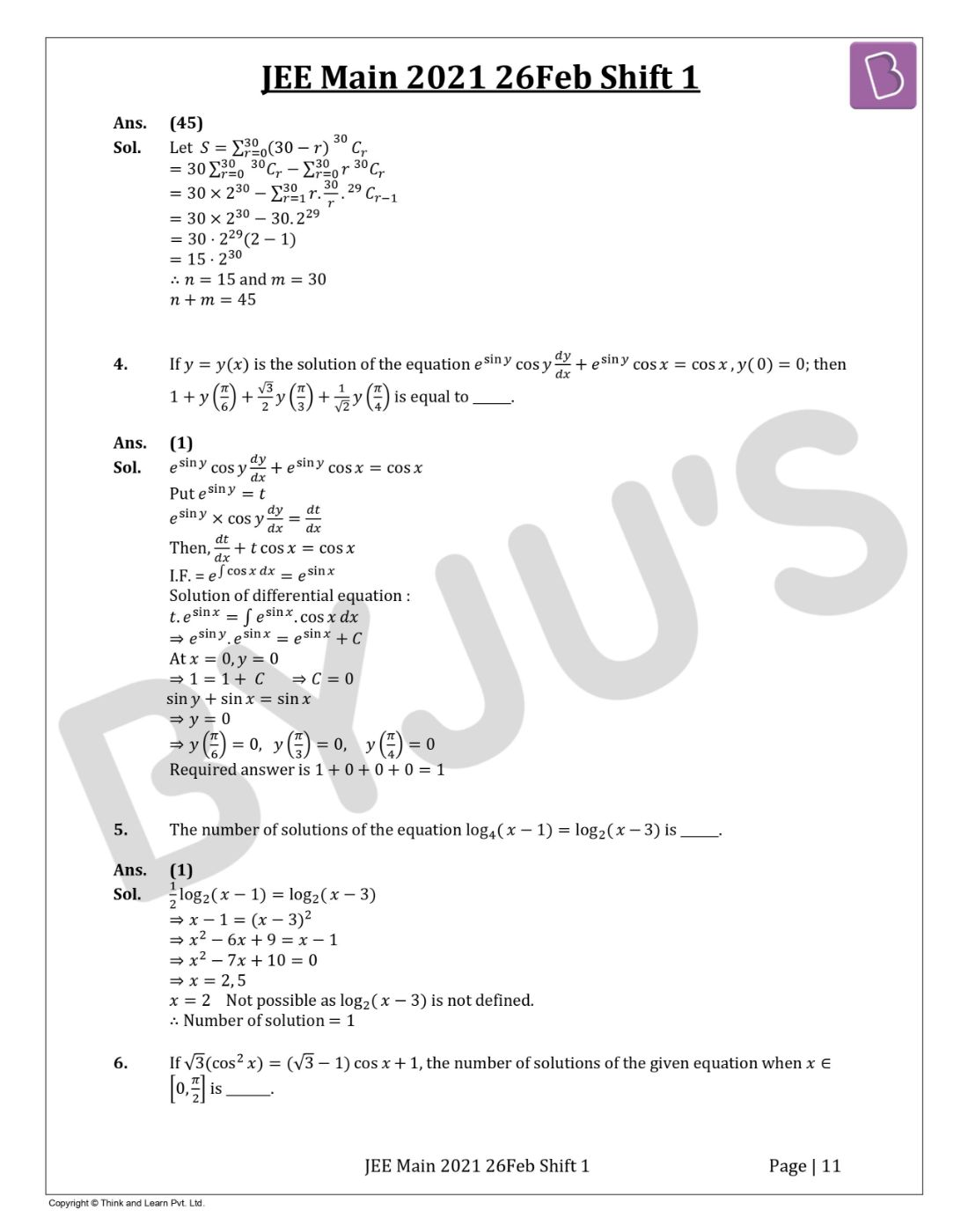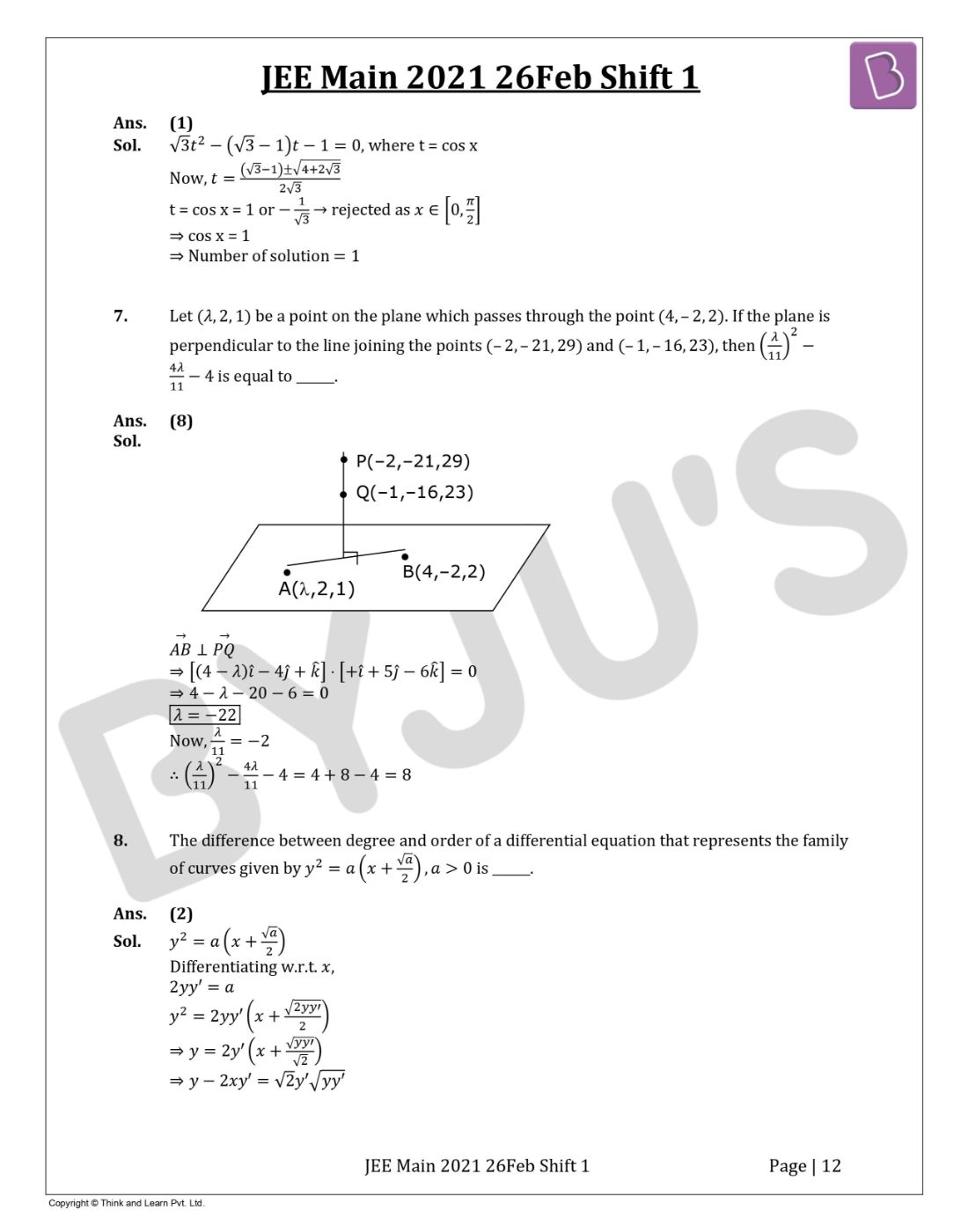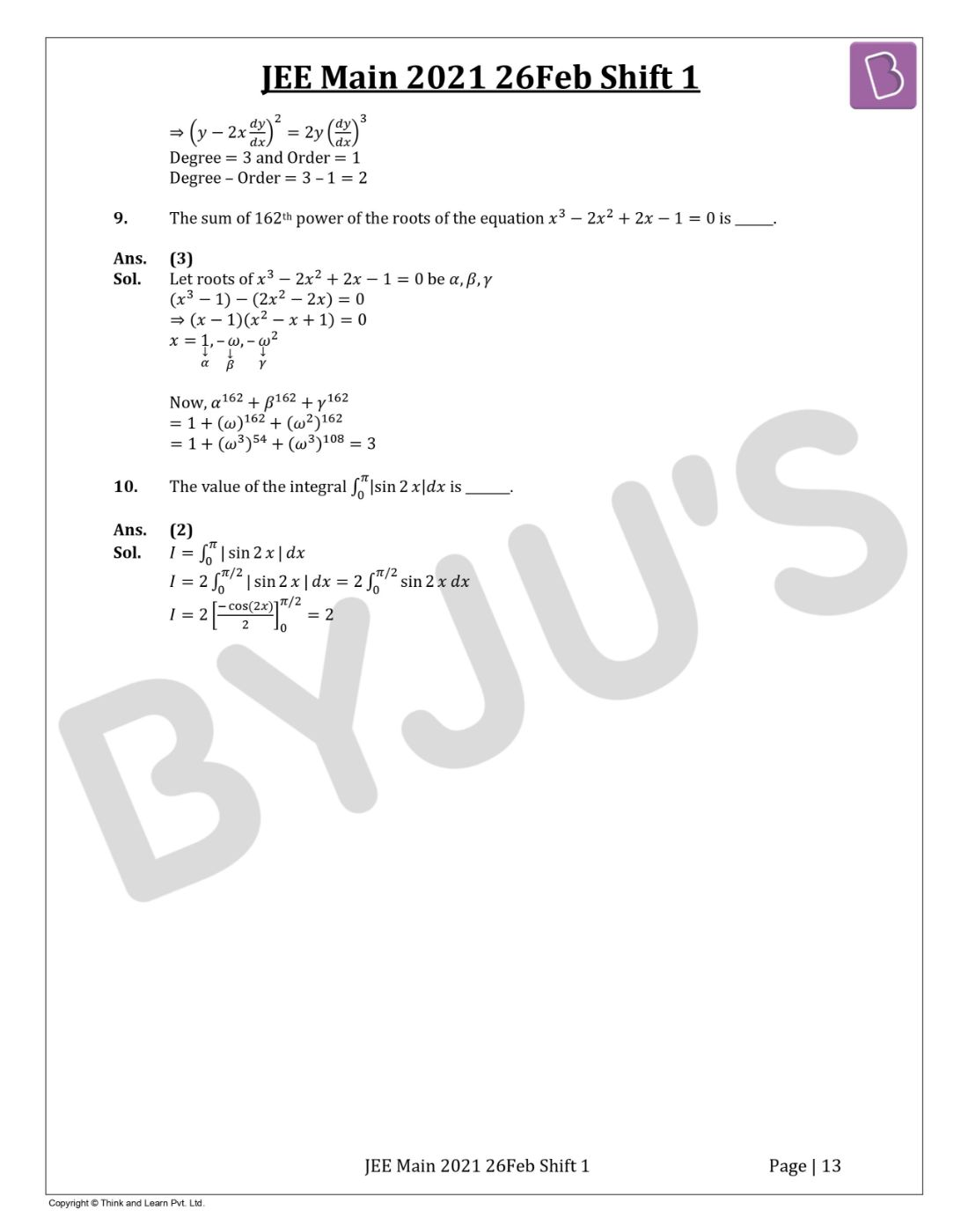Gauge your potential with Aakash BYJU'S JEE Main'23 Mock Test Gauge your potential with Aakash BYJU'S JEE Main'23 Mock Test

# JEE Main 2021 February 26 – Shift 1 Maths Question Paper with Solutions

JEE Main 2021 26th February shift 1 Maths question paper with solutions is available here. Candidates will find memory-based questions of the Maths paper and these will help students crosscheck the correct answers and analyze their performance. All questions asked in JEE Main 2021 paper for Maths (February Shift 1) are solved by subject experts and in a detailed manner. Students can also download the solved paper in a PDF format for offline practice to improve their speed and accuracy.
JEE Main 2021 26th February Shift 1 Maths Question Paper

SECTION A

Question 1: The number of seven-digit integers with the sum of the digits equal to 10 and formed by using the digits 1, 2 and 3 only is:

a. 77
b. 42
c. 35
d. 82

Case – I: 1, 1 , 1, 1, 1, 2, 3

Number of ways = 7! / 5! = 42

Case – II: 1, 1, 1, 1, 2, 2, 2

Number of ways = 7! / [4! * 3!] = 35

Total number of ways = 42 + 35 = 77

Question 2: The maximum value of the term independent of ‘t’ in the expansion of [(tx1/5 + {(1 – x)1/10 / t}]10 where x ∈ (0, 1) is :

a. 10! / [√3 (5!)2]
b. [2 . 10!] / [3 (5!)2]
c. [10!] / [3 (5!)2]
d. [2 . 10!] / [3√3 (5!)2]

Tr+1 = 10Cr (tx1/5)10-r {[(1 – x)1/10] / t}r

= 10Cr t 10-2r x[10-r]/5 (1 – x)r/10

10 – 2r = 0

r = 5

T6 = 10C5 x √1 – x

dT6 / dx = 10C5 [√(1 – x) – (x/2√(1 – x)] = 0

1 – x = x / 2

x = 2 / 3

Max (T6) = {[10!] / [5! 5!] *  / [3√3]}

Question 3: The value of ∑n=1100n-1n ex-[x] dx, where [x] is the greatest integer ≤ x, is :

a. 100 (e – 1)
b. 100e
c. 100 (1 – e)
d. 100 (1 + e)

n=1100n-1n ex-[x] dx

= ∫01 e{x} dx + ∫12 e{x} dx + ∫23 e{x} dx + …….. ∫99100 e{x} dx {because {x} = x – [x]}

= ex |01 + e(x-1)|12 + e(x-2)|23 + …… + e(x-99)|99100

= (e – 1) + (e – 1) + (e – 1) + …… + (e – 1)

= 100 (e – 1)

Question 4: The rate of growth of bacteria in a culture is proportional to the number of bacteria present and the bacteria count is 1000 at initial time t = 0. The number of bacteria has increased by 20% in 2 hours. If the population of bacteria is 2000 after k / loge (6 / 5) hours, then (k / loge 2)2 is equal to :

a. 4
b. 2
c. 16
d. 8

Let x be the number of bacteria at time t.

dx / dt ∝ x

dx / dt = λx

1000x dx / x = ∫0t λ dt

(ln x) – (ln 1000) = λt

ln (x / 1000) = λt

Put t = 2, x = 1200

(ln 12 / 10) = 2λ

λ = (1 / 2) ln (6 / 5)
Now, ln (x / 1000) = (t / 2) ln (6 / 5)

x = 1000et/2 ln (6 / 5)

Given, x = 2000 at t = k / loge (6 / 5)

2000 = 1000 e[k/2 ln (6 / 5)] * [ln (6 / 5)]

2 = ek/2 ⇒ ln 2 = k / 2

k / ln 2 = 2

[k / ln 2]2 = 4

Question 5: If

$$\begin{array}{l}\vec{a}\end{array}$$
and
$$\begin{array}{l}\vec{b}\end{array}$$
are perpendicular, then
$$\begin{array}{l}\vec{a}\times(\vec{a}\times(\vec{a}\times(\vec{a}\times\vec{b})))\end{array}$$
is equal to :

a. (1 / 2) |

$$\begin{array}{l}\vec{a}\end{array}$$
|4
$$\begin{array}{l}\vec{b}\\\end{array}$$

b.
$$\begin{array}{l}\vec{a}\times\vec{b}\\\end{array}$$

c. |
$$\begin{array}{l}\vec{a}\end{array}$$
|4
$$\begin{array}{l}\vec{b}\\\end{array}$$

d.
$$\begin{array}{l}\vec{0}\\\end{array}$$

$$\begin{array}{l}\\\vec{a}\times(\vec{a}\times((\vec{a}.\vec{b})\vec{a}-|\vec{a}|^{2}\vec{b}))\\ =\vec{a}\times(-|\vec{a}|^{2}(\vec{a}\times\vec{b}))\\ =-|\vec{a}|^{2}((\vec{a}.\vec{b})\vec{a}-|\vec{a}|^{2}\vec{b})\\ =-(\vec{a}.\vec{b})\vec{a}|\vec{a}|^{2}+|\vec{a}|^{4}\vec{b}\\ =|\vec{a}|^{4}\vec{b} (\because \vec{a}.\vec{b}=0)\\\end{array}$$

Question 6: In an increasing geometric series, the sum of the second and the sixth term is 25 / 2 and the product of the third and fifth term is 25. Then, the sum of 4th, 6th and 8th terms is equal to :

a. 35
b. 30
c. 26
d. 32

Let a be the first term and r be the common ratio.

ar + ar5 = 25 / 2 and ar2 x ar4 = 25

a2r6 = 25

ar3 = 5

a = 5 / r3 …(1)

(5r / r3) + (5r5 / r3) = 25 / 2

(1 / r2) + r2 = 5 / 2

Put r2 = t

[t2 + 1] / t = 5 / 2

2t2 – 5t + 2 = 0

2t2 – 4t – t + 2 = 0

(2t – 1) (t – 2) = 0

t = 1 / 2, 2

r2 = 1 / 2, 2

r = √2 as the G.P. is increasing.

ar3 + ar5 + ar7

= ar3( 1 + r2 + r4)

= 5 [1 + 2 + 4]

= 35

Question 7: Consider the three planes

P1 : 3x + 15y + 21z = 9,

P2 : x – 3y – z = 5, and

P3 : 2x + 10 y + 14z = 5

Then, which one of the following is true ?

a. P1 and P3 are parallel.
b. P2 and P3 are parallel.
c. P1 and P2 are parallel.
d. P1, P2 and P3 all are parallel.

P1 = x + 5y + 7z = 3

P2 = x – 3y – z = 5

P3 = x + 5y + 7z = 5/2

P1||P3

Question 8: The sum of the infinite series 1 + (2 / 3) + (7 / 32) + (12 / 33) + (17 / 34) + (22 / 35) + …… is equal to :

a. 9 / 4
b. 15 / 4
c. 13 / 4
d. 11 / 4

s = 1 + (2 / 3) + (7 / 32) + (12 / 33) + (17 / 34) + (22 / 35) + ……

(s / 3) = (1 / 3) + (2 / 32) + (7 / 33) + ……. infinity

_________________________________

(2s / 3) = 1 + (1 / 3) + (5 / 32) + (5 / 33) + ….. infinity

2s / 3 = (4 / 3) + (5 / 3) [(1 / 3) / (1 – (1 / 3)] = (4 / 3) + (5 /6) = 13 / 6

s = 13 / 4

Question 9: The value of

$$\begin{array}{l}\begin{bmatrix} (a+1)(a+2)& a+2 & 1\\ (a+2)(a+3)& a+3 & 1\\ (a+3)(a+4)& a+4 & 1 \end{bmatrix}\end{array}$$
is

a. –2
b. (a + 1) (a + 2) (a + 3)
c. 0
d. (a + 2) (a + 3) (a + 4)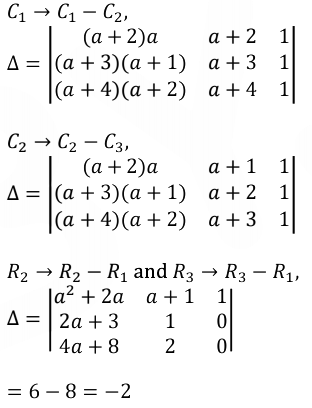Question 10: If (sin-1 x) / a = (cos-1 x) / b) = (tan-1 y) / c; 0 < x < 1, then the value of cos (πc / [a + b]) is:

a. [1 – y2] / 2y
b. [1 – y2] / [1 + y2]
c. 1 – y2
d. [1 – y2] / y √y

(sin-1 x) / a = (cos-1 x) / b) = (tan-1 y) / c

(sin-1 x) / a = (cos-1 x) / b) = [sin-1 x + cos-1 x] / (a + b) = π / [2 (a + b)]

Now, [tan-1 y / c] = π / [2 (a + b)]

2 tan-1 y = (πc / (a + b))

cos (πc / [a + b]) = cos (2 tan-1 y) = [1 – y2] / [1 + y2]

Question 11: Let A be a symmetric matrix of order 2 with integer entries. If the sum of the diagonal elements of A2 is 1, then the possible number of such matrices is :

a.6
b. 1
c. 4
d. 12

Let A =

$$\begin{array}{l}\begin{bmatrix} a &b \\ b&c \end{bmatrix}\\\end{array}$$
$$\begin{array}{l}A^{2}=\begin{bmatrix} a &b \\ b&c \end{bmatrix}\begin{bmatrix} a &b \\ b&c \end{bmatrix}=\begin{bmatrix} a^2+b^2 &ab+bc \\ ab+bc&c^2+b^2 \end{bmatrix}\\\end{array}$$

a2 + 2b2 + c2 = 1

a = 1, b = 0, c = 0

a = 0, b = 0, c = 1

a = –1, b = 0, c = 0

c = –1, b = 0, a = 0

Question 12: The intersection of three lines x – y = 0 and x + 2y = 3 and 2x + y = 6 is a:

a. Equilateral triangle
b. Right angled triangle
c. Isosceles triangle
d. None of the above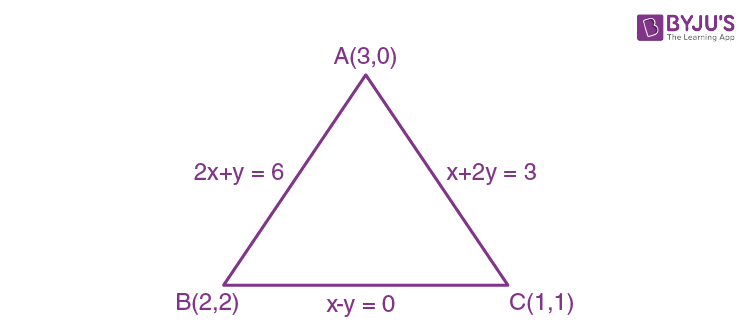AB = AC = √5, BC = √2

Hence, the triangle is isosceles.

Question 13: The maximum slope of the curve y = (1 / 2) x4 – 5x3 + 18x2 – 19x occurs at the point:

a. (2, 9)
b. (2, 2)
c. (3, 21 / 2)
d. (0, 0)

dy / dx = 2x3 – 15x2 + 36x – 19

Let f (x) = 2x3 – 15x2 + 36x – 19

f’ (x) = 6x2 – 30x + 36 = 0

x2 – 5x + 6 = 0

x = 2, 3

f’’ (x) = 12x – 30

f’’ (x) < 0 for x = 2

So, at x = 2, slope is maximum.

y = 8 – 40 + 72 – 38

= 72 – 70

= 2

Maximum slope occurs at (2, 2).

Question 14: Let f be any function defined on R and let it satisfy the condition:

|f (x) – f (y)|≤ |(x – y)2|, ∀ x, y ∈ R. If f (0) = 1, then :

a. f (x) < 0, ∀ x ∈ R
b. f (x) can take any value in R
c. f (x) = 0, ∀ x ∈ R
d. f (x) > 0, ∀ x ∈ R

|f (x) – f (y)| ≤ |(x – y)2|, ∀ x, y ∈ R

|[f (x) – f (y)] / [(x – y)]| ≤ |x – y|

limx→y |[f (x) – f (y)] / [(x – y)]| ≤ 0

|f’ (y)| ≤ 0

f’ (y) = 0

f (y) = c

Since f (0) = 1, f (y) = 1, ∀ y ∈ R

Question 15: The value of ∫-π/2π/2 cos2 x / [1 + 3x] dx is:

a. 2π
b. 4π
c. π / 2
d. π / 4

Let I = ∫-π/2π/2 cos2 x / [1 + 3x] dx

I = ∫-π/2π/2 [cos2 x] / [1 + 3-x] dx

= ∫-π/2π/2 [3x cos2 x] / [1 + 3x] dx

2I = ∫-π/2π/2 cos2 x dx

I = ∫0π/2 cos2 x dx

= π / 4

Question 16: The value of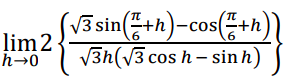is :

a. 3 / 4
b. 2 / √3
c. 4 / 3
d. 2 / 3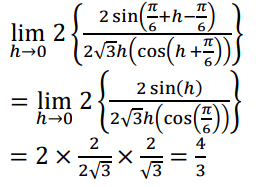Question 17: A fair coin is tossed a fixed number of times. If the probability of getting 7 heads is equal to the probability of getting 9 heads, then the probability of getting 2 heads is:

a. 15 / 212
b. 15 / 213
c. 15 / 214
d. 15 / 28

P (x = 9) = P (x = 7)

nC9 (1 / 2)n-9 (1 / 2)9 = nC7 (1 / 2)n-7 (1 / 2)7

nC9 (1 / 2)n = (1 / 2)n x nC7

⇒ n = 9 + 7 = 16

P (x = 2) = 16C2 (1 / 2)14 (1 / 2)2

= 16C2 (1 / 2)16

= 15 / 213

Question 18: If (1, 5, 35), (7, 5, 5), (1, λ, 7) and (2 λ, 1, 2) are coplanar, then the sum of all possible values of λ is:

a. − 44 / 5
a. 39 / 5
a. − 39 / 5
a. 44 / 5

Let P (1, 5, 35), Q (7, 5, 5), R (1, λ, 7) and S (2 λ, 1, 2)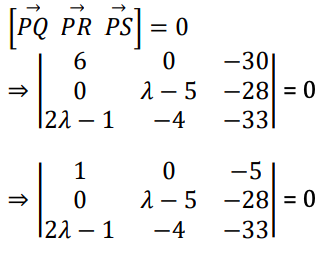⇒ {−33λ + 165 − 112} + 5(λ − 5)(2λ − 1) = 0

⇒ 53 − 33λ + 5 {2λ2 − 11λ + 5} = 0

⇒ 10λ2 − 88λ + 78 = 0

2 − 44λ + 39 = 0

∴ λ1 + λ2 = 44 / 5

Question 19: Let R = {P,Q)|P and Q are at the same distance from the origin} be a relation, then the equivalence class of (1,–1) is the set :

a. S = {(x, y)|x2 + y2 = 1}
b. S = {(x, y)|x2 + y2 = 4}
c. S = {(x, y)|x2 + y2 = √2}
d. S = {(x, y)|x2 + y2 = 2}

P(a, b) , Q(c, d), OP = OQ

a2 + b2 = c2 + d2

R(x ,y), S = (1, –1) OR = OS ( equivalence class)

x2 + y2 = 2

Question 20: In the circle given below, let OA = 1 unit, OB = 13 unit and PQ perpendicular to OB. Then, the area of the triangle PQB (in square units) is :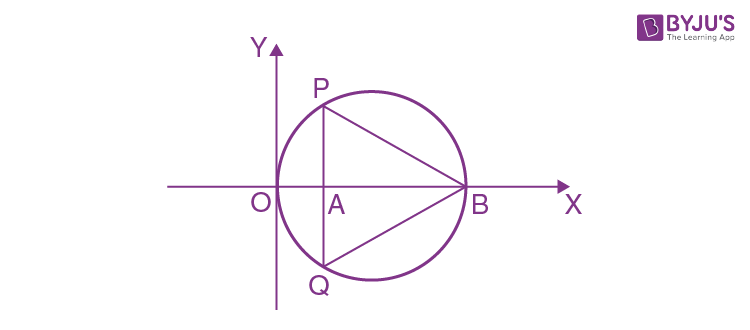a. 26 √3
b. 24 √2
c. 24 √3
d. 26 √2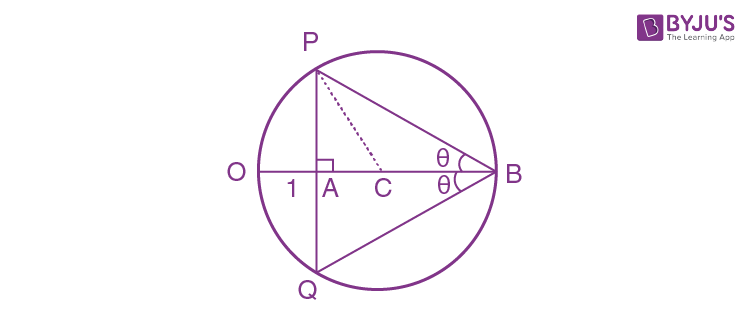OC = 13 / 2 = 6.5

AC = CO – AO

= 6.5 – 1

= 5.5

In △PAC

PA = √(6.52 – 5.52)

PA = √12

PQ = 2PA = 2 √12

Now, area of △PQB = (1 / 2) * PQ * AB

= (1 / 2) * 2 √12 * 12

= 12 √12

= 24 √3

Section-B

Question 1: The area bounded by the lines y = |x – 1| – 2 is ______.

NTA Ans. (8)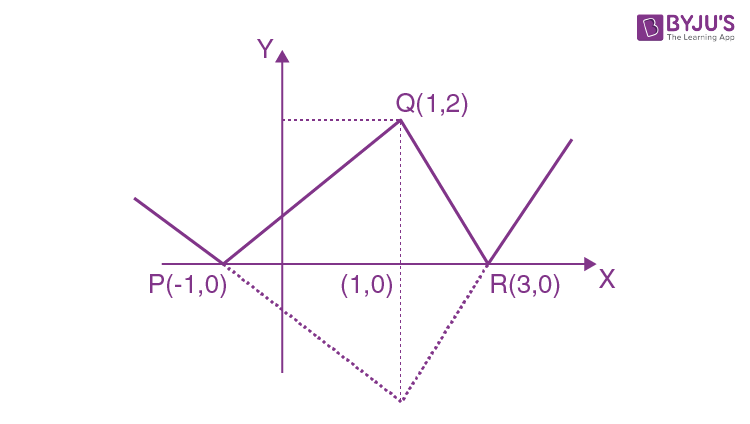Required area area of △PQR

Area = (1 / 2) * 4 * 2 = 4

This is a bonus question as the second curve is also not given.

If the second curve is x – axis, then answer will be 4

Question 2: The number of integral values of ‘k’ for which the equation 3 sin x + 4 cos x = k + 1 has a solution, k ∈ R is ______.

3 sin x + 4 cos x = k + 1

⇒ −5 ≤ k + 1 ≤ 5

⇒ −6 ≤ k ≤ 4

−6, −5, −4, −3, −2, −1, 0, 1, 2, 3, 4 → 11 integral values

Question 3: Let m, n ∈ N and gcd (2, n) = 1. If 30 30C0 + 29 30C1 + ….. + 2 30C28 + 1 30C29 = n . 2m, then n + m =

Let S = ∑r=030 (30 – r) 30Cr

= 30 ∑r=03030Cr – ∑r=030 r 30Cr

= 30 * 230 – ∑r=130 r (30 / r) 29Cr-1

= 30 * 230 – 30 * 229

= 30 * 229 (2 – 1)

= 15 * 230

n = 15 and m = 30

n + m = 45

Question 4: If y = y (x) is the solution of the equation esiny cosy (dy / dx) + esiny cosx = cos x, y (0) = 0; then 1 + y (π / 6) + (√3 / 2) y (π / 3) + (1 / √2) y (π / 4) is equal to ______.

esiny cosy (dy / dx) + esiny cosx = cos x

Put esiny = t

esiny * cos y (dy / dx) = dt / dx

Then, dt / dx + t cos x = cos x

IF = e∫cosx dx = esinx

Solution of differential equation

t . esinx = ∫esinx cosx dx

esiny . esinx = esinx + c

At x = 0, y = 0

1 = 1 + c ⇒ c = 0

sin y + sin x = sin x

y = 0

y (π / 6) = 0, y (π / 3) = 0, y (π / 4) = 0

The required answer is 1 + 0 + 0 + 0 = 1.

Question 5: The number of solutions of the equation log4 (x – 1) = log2 (x – 3) is ______.

(1 / 2) log2 (x − 1) = log2 (x − 3)

⇒ x − 1 = (x − 3)2

⇒ x2 − 6𝑥 + 9 = x − 1

⇒ x2 − 7𝑥 + 10 = 0

⇒ x = 2, 5

x = 2 Not possible as log2 (x − 3) is not defined.

∴ Number of solution = 1

Question 6: If √3 (cos2 x) = (√3 – 1) cos x + 1 the number of solutions of the given equation when x ∈ [0, π / 2] is _______.

√3 t2 – (√3 – 1) t – 1 = 0, where t = cos x

Now, t = {(√3 – 1) ± [√4 + 2 √3]} / 2 √3

t = cos x = 1 or – 1 / √3 (rejected as x ∈ [0, π / 2])

cos x = 1

Number of solution = 1

Question 7: Let (λ, 2, 1) be a point on the plane which passes through the point (4, – 2, 2). If the plane is perpendicular to the line joining the points (– 2, – 21, 29) and (– 1, – 16, 23), then (λ / 11)2 – (4λ / 11) – 4 is equal to ______.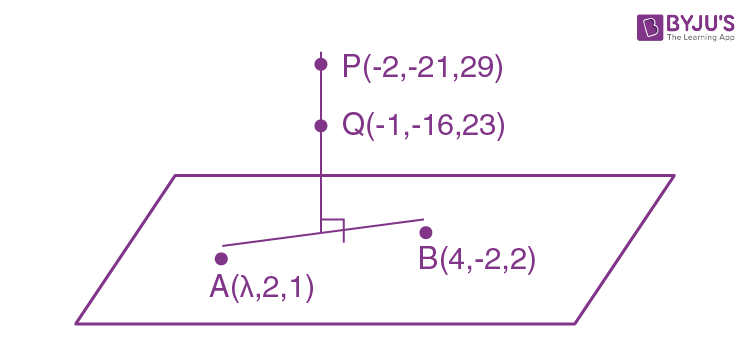AB is perpendicular to PQ.

[(4 – λ) i – 4j + k] . [i + 5j – 6k] = 0

4 – λ – 20 – 6 = 0

λ = – 22

Now, (λ / 11) = – 2

(λ / 11)2 – (4λ / 11) – 4 = 4 + 8 – 4 = 8

Question 8: The difference between degree and order of a differential equation that represents the family of curves given by y2 = a (x + (√a / 2)), a > 0 is ______.

y2 = a (x + (√a / 2))

Differentiating w.r.t.

2yy’ = a

y2 = 2yy’ [x + (√2yy’ / 2)]

y = 2y’ [(x + √yy’ / 2)]

y – 2xy’ = √2y’ √yy’

[y – 2x (dy / dx)]2 = 2y (dy / dx)3

Degree = 3 and Order = 1

Degree – Order = 3 – 1 = 2

Question 9: The sum of 162th power of the roots of the equation x3 – 2x2 + 2x – 1 = 0 is _________.

Let roots of x3 – 2x2 + 2x – 1 = 0 be ɑ, β, γ.

(x3 – 1) – (2x2 – 2x) = 0

(x – 1) (x2 – x + 1) = 0

x = 1, – 𝞈, – 𝞈2

Now, ɑ162 + β162 + γ162

= 1 + (𝞈)162 + (𝞈2)162

= 1 + (𝞈3)54 + (𝞈3)108 = 3

Question 10: The value of the integral ∫0π |sin 2x| dx is _______.

I = ∫0π |sin 2x| dx

= 2 ∫0π/2 |sin 2x| dx = 2 ∫0π/2 sin 2x dx

= 2 [- cos (2x) / 2]0π/2

= 2